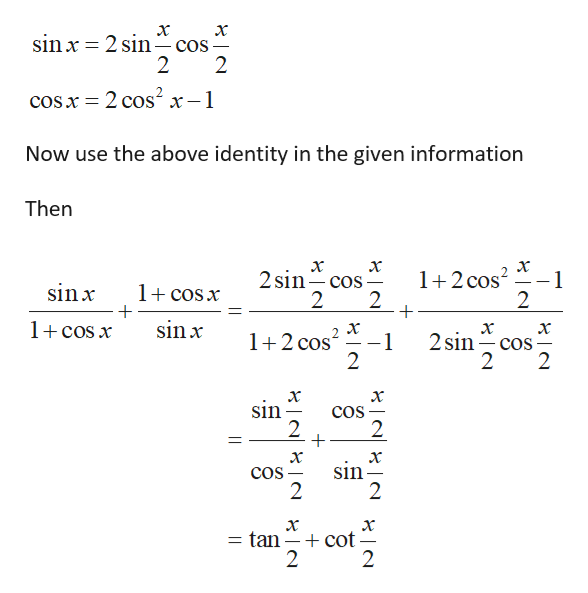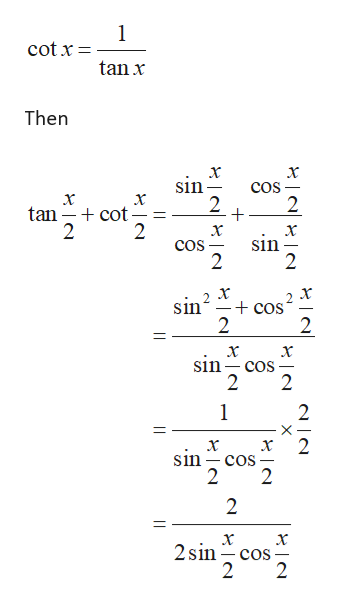# Perform the addition and use the fundamental identities to simplify.sin x / (1+cos x)   +   (1+cos x) / sin x

Question
2 views

Perform the addition and use the fundamental identities to simplify.

sin x / (1+cos x)   +   (1+cos x) / sin x

check_circle

star
star
star
star
star
1 Rating
Step 1

Consider the given information

Step 2

Now using the half angle identityhelp_outlineImage Transcriptioncloseх sin x = 2 sin- cos- 2 cos x = 2 cos? x-1 Now use the above identity in the given information Then х х 2 sin- cos х 1+2 cos sin x 1+ cosx 1+ cos x sin x х х 2 sin- cos - 2 х 1+2 cos -1 2 2 sin cos - 2 2 sin cos - х + cot = tan || fullscreen
Step 3

Now using the i...help_outlineImage Transcriptionclosecot x = tan x Then х cos sin х х + cot - tan sin 2 cos sin + cos sin cos 2 sin cos 2 sin cos fullscreen

### Want to see the full answer?

See Solution

#### Want to see this answer and more?

Solutions are written by subject experts who are available 24/7. Questions are typically answered within 1 hour.*

See Solution
*Response times may vary by subject and question.
Tagged in

### Trigonometry